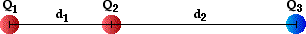# Electricity: Static Electricity

## Static Electricity: Audio Guided Solution

#### Problem 24:

Consider the diagram below.GIVEN: Q1 = +5.00x10-7 C; Q2 = +4.00x10-7 C; Q3 = -8.00x10-7 C; d1 = 5.00 cm; d2 = 8.00 cm.
a. Determine the magnitude and direction of the force exerted by Q2 upon Q1.
b. Determine the magnitude and direction of the force exerted by Q3 upon Q1.
c. Determine the magnitude and direction of the net electric force on Q1.

## Audio Guided Solution

Click to show or hide the answer!

## Habits of an Effective Problem Solver

• Read the problem carefully and develop a mental picture of the physical situation. If necessary, sketch a simple diagram of the physical situation to help you visualize it.
• Identify the known and unknown quantities; record them in an organized manner. A diagram is a great place to record such information. Equate given values to the symbols used to represent the corresponding quantity - e.g., Q1 = 2.4 μC; Q2 = 3.8 μC; d = 1.8 m; Felect = ???.
• Use physics formulas and conceptual reasoning to plot a strategy for solving for the unknown quantity.
• Identify the appropriate formula(s) to use.
• Perform substitutions and algebraic manipulations in order to solve for the unknown quantity.